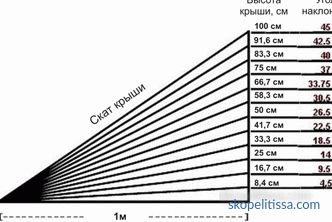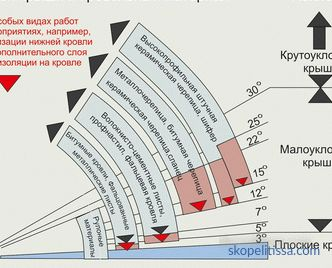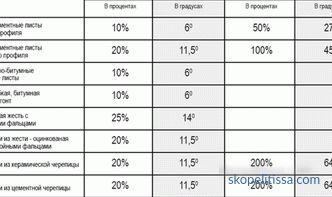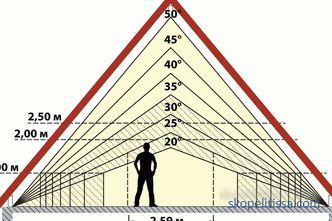# How to calculate the angle of the roof with examples

Roofs of buildings and structures are divided into two categories: flat and inclined. This article will deal with the second position, or rather, with the angle of inclination of the roof: which characteristics affect this parameter, which roofing materials at what angle of the roof fit, in what units the angle of inclination of the skates changes. Having understood the information received, you can easily monitor the correctness of the construction of the roof of your house.

## Units of Measurement of the Angle of the Slope

From the school geometry course everyone knows that the magnitude of any Angle is measured in degrees. The slope of the roof in this case is no exception. But it is necessary to designate that in technical literature, in State Standards and reference books other units of measurement are also encountered. Namely: interest and relations of the parties.

First of all, it is necessary to indicate what is meant by the angle of the roof slope. In the photo below it is designated by the Latin letter alpha.

In the construction sphere of the roof with an angle of inclination above 50 ° are rare. These include turrets in the Gothic style or the lower slopes of mansard roofs. Basically the maximum parameter is 45 °.

As for the two other units of measurement, the ratio of the sides is the so-called simplified fraction. It is based on two sizes: the height of the roof, in the photo it is marked with the letter "H", and the projection of the slope, indicated by the letter "L". The ratio should be: H / L.

We add that the projection of the length of the slope is, in fact, half the width of the house, if the roof is symmetrical, or full width, if the roof is single-sided. In this case, the angle of inclination is written with a fraction, for example, 1: 3.

In some designs, the designation of a fraction is inconvenient. For example, if it looks like this: 4:13. Therefore, use the percentage calculation. It is calculated as follows:

4: 13x100 = 30.77%

The roof slope in percentages and degrees is used by professional builders more often than the ratio of the sides, because this designation used in reference books and technical literature. That is, these are purely technical units. Although it is not difficult to convert from one unit to another. The photo below shows the translation from degrees and attitudes of the sides and vice versa.The proportions of the ratio of degrees to roof dimensions

If there is a need to convert degrees to percents, then, based on the figure above, you can do several mathematical calculations. An angle equal to 45 ° is taken as 100%. Now you can find how many degrees are in one percent. For this you need:

45/100 = 0.45 °. We translate into minutes, it turns out 27 '. That is, 1% is 27 degree minutes.

You can approach the solution of the problem from the reverse side. That is, to convert degrees to percentages. Here, the inverse ratio:

100/45 = 2.22%

It turns out that in one degree 2.22%.

On our website you can find contacts of construction companies that offer the service of calculating, installing and repairing roofing. Directly to communicate with representatives, you can visit the exhibition of houses "Low-rise Country".

## The dependence of the slope of the slope and the type of roofing material

I already mentioned two types of roofing: flat and inclined. But it should be noted that flat roofs also have their own angle, which varies in the range of 0-5 °. Pitched roofs are divided into two subgroups (division conditional):

• with a slight slope - from 6 to 30 °;

• with a steep slope - more 30 °.The distribution of roofing materials by the slope of the slope

Each type of roof construction has its own advantages and disadvantages. For example, flat - this is a small area of ​​coverage, which saves on roofing material (its quantity). But at the same time such a construction would require reinforced waterproofing. Steep slopes - this is a smooth convergence of precipitation, but the high windage of the structure, which will require from its construction to strengthen the truss system.

Given this, it is necessary to strictly approach the choice of roofing material. Their manufacturers must inform consumers, at what minimum slope of the roof they can be used. The photo below shows a diagram of the distribution of roofing materials at the corners of ramps.

As can be seen from the diagram, rolled materials in the form of roofing material or roofing felts are usually used on flat roofs. Although there are no contraindications to the use of sheet or plate products: slate, corrugated sheet metal, sheet metal, folded. But a piece of roofing material is better on roofs with a slope less than 15 ° not to use. There is a high probability of water penetration through the joints of the coating elements.Roof Angle Value in degrees and percent relative to the type of roofing material

## Roof design features depending on the angle of inclination

If the draft is the slope of the roof, then calculate its height is not difficult. And this parameter is one of the most important, because it determines the height of the skate. This element of the roof structure is the point of departure in the roof structure as a whole. Because during the construction, they first set the level of the ridge, and already cut lumber under it: support stands. From here also calculate the length of the truss legs.

The complexity of the calculation lies in the fact that not everyone remembers trigonometry, whose formulas are used to calculate the lengths of the elements of the roof structure. The basis of the formulas are trigonometric functions: sine, cosine, tangent, catangent.

For example, how to determine the height of the ridge (respectively, and the roof) through the sine and tangent:

sin α = H / S, where "S" is the length of the slope. Accordingly, the height of the ridge will be equal to: H = S x sin α.

tg α = H / L , that is, H = l x tg α

Similarly, you can determine the length of the slope, or, knowing both parameters, You can calculate the angle of the roof. All design parameters are interrelated, so that, knowing two of them, you can determine the third.

It may be interesting! In the article on the following link read about the ventilation of the under-roof space.

By the way, determining the roof parameters, you can do without the angle of inclination. For this, the Pythagorean formula is used. Here is its formula:

S 2 = H 2 + L 2

Swapping the values, the desired value is found.

You may have a question related to the value of the values ​​of trigonometric functions. They are freely available, but so that you do not search for them on the Internet and spend your time, we offer the following table:

We add that trigonometric functions simplify the calculations of roof structures. If you use them correctly and be able to sketch sketches of roofs on paper (having imagination in terms of the constructive arrangement of its elements), then you can easily calculate the dimensions of each element.

This applies not only to single-pitch or gable structures. It is also easy to calculate the elements of hip or mansard roofs. Just have to break their complex structures into simple ones.

## The influence of the angle of inclination on the volume of the room of the attic

If the house is erected with an attic, the angle of inclination of the skates acquires an applied value. And here the ratio is direct, that is, the greater the angle, the greater the volume of the attic. To be clear, the photo below is clearly visible.Ratio of slope angle to volume of mansard room

Let us give an example based on the upper figure. Here you can clearly see that the height of the room depends on the parameters of the slope of the roof. It should be borne in mind that the optimum height should not be less than 2.5 m. This means that the angle of slope of the skylights should be at least 25 °. But note that with this arrangement of the elements of the roof is not taken into account the volume of the room. That is, the width of the room will not be more than 3 m. Moreover, the width of the house is 10 m. It turns out that only 30% of the space is used.

So, it is necessary to increase the value of the angle. And the more it is, the more useful space of the attic. But there is a reverse side of the coin. Increasing the angle increases the height of the ridge. And this is the cost of building materials for the construction of the roof, plus an increase in the sail structure. Therefore, it is very important to find a middle ground. For example, 40 ° practically solve all problems.

To get away from these problems once and for all, builders have long used a completely different design. It is difficult both in the construction and in the calculations, but this option solves the problem of the usable floor space without increasing the height of the roof.